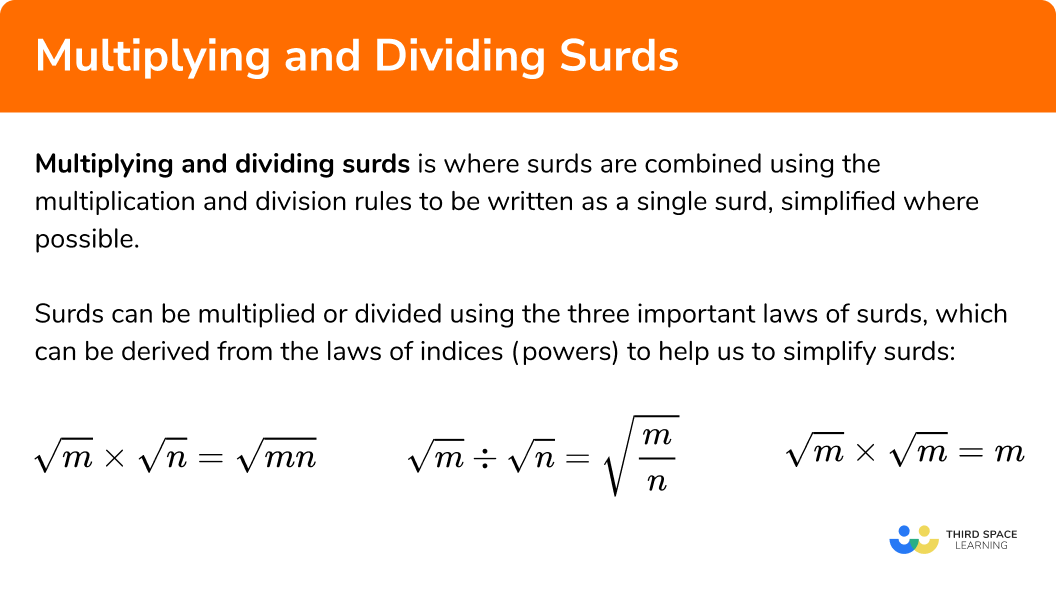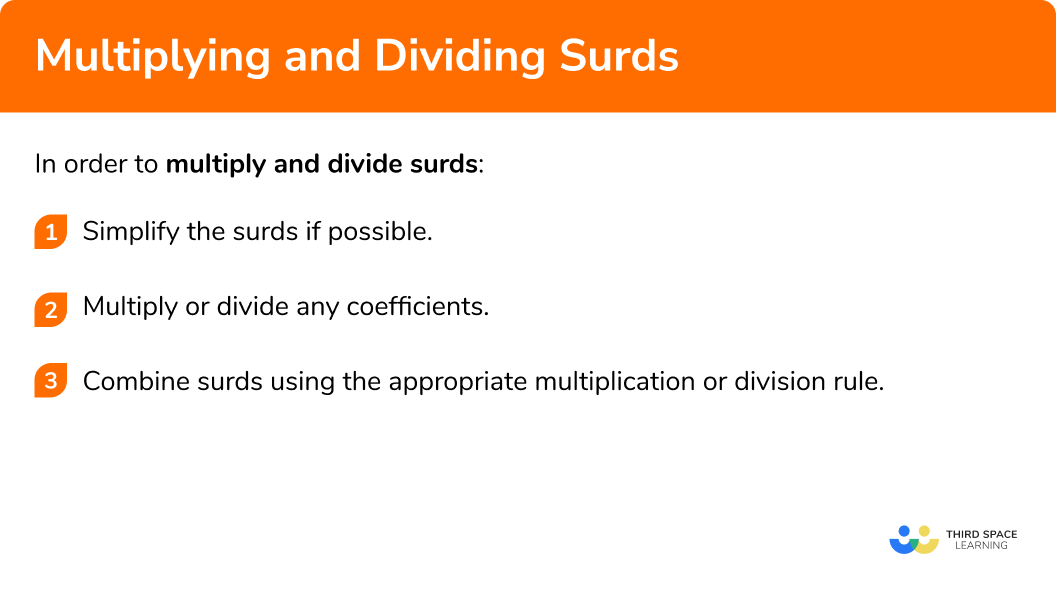GCSE Maths Number Surds

Multiplying And Dividing Surds

# Multiplying And Dividing Surds

Here we will learn about multiplying and dividing surds including when surd expressions can be multiplied or divided, and how to carry out these calculations. You’ll also learn how to expand single and double brackets involving surds.

There are also multiplying and dividing surds worksheets based on Edexcel, AQA and OCR exam questions, along with further guidance on where to go next if you’re still stuck.

## What is multiplying and dividing surds?

Multiplying and dividing surds is where surds are combined using the multiplication and division rules to be written as a single surd, simplified where possible.

Surds can be multiplied or divided using the three important laws of surds, which can be derived from the laws of indices (powers) to help us to simplify surds:

\begin{aligned} &\sqrt{m} \times \sqrt{n}=\sqrt{m n} \\\\ &\sqrt{m} \div \sqrt{n}=\sqrt{\frac{m}{n}} \\\\ &\sqrt{m} \times \sqrt{m}=m \end{aligned}

You may be asked to apply these skills at GCSE maths to give answers to problems in geometry, such as Pythagoras or trigonometry, as exact values, rather than as decimals. Before calculators were invented, surds were the standard form for stating answers which were irrational numbers.

The formula for solving quadratics also uses a square root sign, so you may need to apply your knowledge of surds here as well. You may also be asked to expand double brackets (binomial expansion) involving surds.

### What is multiplying and dividing surds?## How to multiply and divide surds

In order to multiply and divide surds:

1. Simplify the surds if possible.
2. Multiply or divide any coefficients.
3. Combine surds using the appropriate multiplication or division rule.

### Explain how to multiply and divide surds## What is multiplying surds?

To multiply surds, simply multiply the numbers outside of the square root sign together and then multiply the square roots together. Finally, simplify if possible.

For example: a\sqrt{b} \times c\sqrt{d} = ac\sqrt{bd}

## What is dividing surds?

To divide surds, divide the whole numbers outside of the square root sign by each other and then divide the square roots by each other. Finally, simplify if possible.

For example: a\sqrt{b} \div c\sqrt{d} = \cfrac{a}{c} \sqrt{\cfrac{b}{d}}

## Multiplying and dividing surds examples

### Example 1: simple multiplication

2 \sqrt{2} \times 3 \sqrt{5}

1. Simplify the surds if possible

2Multiply or divide any coefficients

2 \times 3=6

3Combine surds using the appropriate multiplication or division rule

\sqrt{2} \times \sqrt{5}=\sqrt{10}

6\sqrt{10}

### Example 2: simple division

\frac{4 \sqrt{15}}{2 \sqrt{5}}

Simplify the surds if possible.

Multiply or divide any coefficients.

Combine surds using the appropriate multiplication or division rule.

### Example 3: a squared surd

(\sqrt{6})^{2}

Simplify the surds if possible.

Multiply or divide any coefficients.

Combine surds using the appropriate multiplication or division rule.

### Practice multiplying and dividing surds questions

1. Simplify

\sqrt{5} \times \sqrt{2}

\sqrt{7}\sqrt{10}5 \sqrt{2}2 \sqrt{5}Using the multiplication rule of surds, we combine into a single surd by multiplying 5 by 2 .

2. Simplify

\sqrt{3} \times \sqrt{7}

3 \sqrt{7}\sqrt{10}2 \sqrt{5}\sqrt{21}Using the multiplication rule of surds, we combine into a single surd by multiplying 3 by 7 .

3. Simplify

\frac{\sqrt{40}}{\sqrt{4}}

\sqrt{10}\sqrt{36}2 \sqrt{5}\sqrt{160}Using the division rule of surds, we combine into a single surd by dividing 40 by 4 .

4. Simplify

\frac{\sqrt{90}}{\sqrt{2}}

5 \sqrt{3}3 \sqrt{5}\sqrt{45}\sqrt{88}Using the division rule of surds, we combine into a single surd by dividing 90 by 2 . Root 45 will then simplify.

5. Simplify fully

\frac{\sqrt{63}}{(\sqrt{3})^{2}}

3 \sqrt{7}2 \sqrt{7}\sqrt{7}\sqrt{21}Using the square rule of surds, (\sqrt{3})^{2}=3 .

The numerator simplifies to 3 \sqrt{7} and finally 3 can be cancelled from the top and bottom of the fraction.

## Simplifying fractions involving surds

Sometimes, a surd division will be written as a fraction, and you may need to simplify the numerator, denominator, or both, before carrying out the division calculation.

This topic is extended further in rationalising the denominator.

## How to simplify fractions involving surds

In order to simplify fractions involving surds:

1. Simplify the surds if possible.
2. Use surd laws to fully simplify the numerator and denominator of the fraction.
3. Divide the numerator by the denominator.

## Simplifying fractions involving surds examples

### Example 4: simplifying fractions involving surds

\frac{2 \sqrt{5} \times 3 \sqrt{6}}{2 \sqrt{2}}

Simplify the surds if possible.

Use surd laws to fully simplify the numerator and denominator of the fraction.

Divide the numerator by the denominator.

### Example 5: simplifying fractions involving surds

\frac{2 \sqrt{48} \times 5 \sqrt{3}}{\sqrt{12}}

Simplify the surds if possible.

Use surd laws to fully simplify the numerator and denominator of the fraction.

Divide the numerator by the denominator.

### Example 6: simplifying fractions involving addition and subtraction of surds

\frac{4 \sqrt{15}+\sqrt{60}}{3\sqrt{5}}

Simplify the surds if possible.

Use surd laws to fully simplify the numerator and denominator of the fraction.

Combine surds using the appropriate multiplication or division rule.

### Practice simplifying fractions involving surds questions

1. Simplify

\frac{\sqrt{15} \times \sqrt{2}}{\sqrt{3}}

\sqrt{10}\sqrt{27}2 \sqrt{5}\sqrt{5}Using the multiplication rule of surds, we combine the numerator into a single surd by multiplying 15 by 2 . We then use the division rule and divide [/katex] 30 [/katex] by 3 to get 10.

2. Simplify

\frac{4 \sqrt{20} \times \sqrt{3}}{2 \sqrt{3}}

2\sqrt{5}4\sqrt{5}8\sqrt{15}2\sqrt{15}The numerator simplifies to 8\sqrt{15} .

Using the division rule, we divide the coefficients (8 \div 2=4) and the surds (15 \div 3=5) to get the answer.

3. Simplify

\frac{\sqrt{24}+\sqrt{96}}{\sqrt{3}}

6\sqrt{3}6\sqrt{6}\sqrt{8}+\sqrt{32}6\sqrt{2}The numerator simplifies to 6\sqrt{6} .

Using the division rule, we divide the surds (6 \div 3=2) to get the answer.

## Expanding single brackets involving surds

You can expand brackets for expressions involving surds, just as you would for algebraic expressions. Just multiply everything inside the bracket by the term outside the bracket.

## How to expand single brackets involving surds

In order to expand brackets involving surds:

1. Simplify the surds if possible.
2. Multiply each term inside the bracket by the term outside.
3. Check and simplify the answer further if possible.

## Expanding single brackets involving surds examples

### Example 7: expanding a single bracket

2(4-3 \sqrt{5})

Simplify the surds if possible.

Multiply each term inside the bracket by the term outside.

Check and simplify the answer further if possible.

### Example 8: expanding a more difficult single bracket

4(\sqrt{5}+2 \sqrt{24})

Simplify the surds if possible.

Multiply each term inside the bracket by the term outside

Check and simplify the answer further if possible

## How to expand double brackets involving surds

In order to expand brackets involving surds:

1. Simplify the surds if possible.
2. Multiply each term inside the first bracket by each term inside the second bracket.
3. Collect like terms and simplify the answer.

### Example 9: expanding double brackets

(2+4 \sqrt{2})(5+2 \sqrt{3})

Simplify the surds if possible.

Multiply each term inside the first bracket by each term inside the second bracket

Collect like terms and simplify the answer

### Example 10: expanding double brackets and simplifying

(6-\sqrt{12})(2 \sqrt{3}+1)

Simplify the surds if possible

Multiply each term inside the first bracket by each term inside the second bracket

Collect like terms and simplify the answer

### Example 11: difference of two squares

(4+5 \sqrt{2})(4-5 \sqrt{2})

Simplify the surds if possible

Multiply each term inside the first bracket by each term inside the second bracket

Collect like terms and simplify the answer

### Practice expanding brackets involving surds questions

1. Simplify

6(4+\sqrt{5})

24+\sqrt{5}24+6 \sqrt{5}24+\sqrt{30}24+3 \sqrt{5}Multiply each term inside the bracket by the 6 outside.

2. Simplify

\sqrt{2}(\sqrt{8}-3)

4-3 \sqrt{2}\sqrt{10}- 3\sqrt{2}16-3\sqrt{2}\sqrt{10}Multiply each term inside the bracket by the root 2 outside, then the square root of 16 is 4.

3. Simplify

(\sqrt{2}+4)(5-\sqrt{2})

\sqrt{2}9 \sqrt{2}16+\sqrt{2}18+\sqrt{2}Multiply each term inside the first bracket by each term inside the second bracket. You should get four terms, then simplify by collecting the integers and the like surds.

4. (5+\sqrt{12})^{2}

25 \sqrt{12}3737+20 \sqrt{3}25+4 \sqrt{3}When a bracket is squared, you need to multiply the bracket by itself, i.e.

(5+\sqrt{12})(5+\sqrt{12})

Expand the double brackets by multiplying each term inside the first bracket by each term inside the second bracket. You should get four terms, then simplify by collecting the integers and the like surds.

5. (4+\sqrt{10})(4-\sqrt{10})

2666+8 \sqrt{10}26-8 \sqrt{10}This is a difference of two squares. Expand the double brackets by multiplying each term inside the first bracket by each term inside the second bracket.

You should get four terms – however, when you simplify, the surd terms will cancel each other out, leaving you with 16-10=6 as the final answer.

### Common misconceptions

• Don’t mix up addition and multiplication laws
\begin{aligned} &\sqrt{3}+\sqrt{3}=2 \sqrt{3} \; \color{green} ✔\\\\ &\sqrt{3} \times \sqrt{3}=\sqrt{9}=3 \; \color{green} ✔ \end{aligned}

Refer back to knowledge of algebra to help; this is like:

a+a=2a and a \times a=a^2 .

• When expanding brackets, make sure both terms inside the bracket have been multiplied by the term outside

3(4 \sqrt{7}-12)=12 \sqrt{7}-12 \; \color{red} ✘

This is incorrect, because the -12 inside the bracket also needs to be multiplied by the 3outside the bracket.

3(4 \sqrt{7}-12)=12 \sqrt{7}-36 \; \color{green} ✔

• When expanding brackets, make sure that the numbers underneath the root symbols aren’t accidentally multiplied by the term outside the bracket

2(3 \sqrt{7}-5)=6 \sqrt{14}-10 \; \color{red} ✘

This is incorrect, because the 7 underneath the square root sign should not have been multiplied by the 2 outside the bracket (to give the incorrect 14 ).

2(3 \sqrt{7}-5)=6 \sqrt{7}-10 \; \color{green} ✔

• Trying to combine unlike surds.

It’s OK to leave an answer with more than one surd in it, if it will not simplify further.

Multiplying and dividing surds is part of our series of lessons to support revision on surds. You may find it helpful to start with the main surds lesson for a summary of what to expect, or use the step by step guides below for further detail on individual topics. Other lessons in this series include:

### Multiplying and dividing surds GCSE questions

1. (a) Simplify fully: \sqrt{32} \times \sqrt{5}

(b) Simplify fully: \frac{\sqrt{90}}{\sqrt{2}}

(2 marks)

(a)

\sqrt{32}=4\sqrt{2}

(1)

4\sqrt{2} \times \sqrt{5}=4\sqrt{10}

(1)

(b)

\sqrt{90}\div \sqrt{2} = \sqrt{45}

(1)

\sqrt{45} = 3\sqrt{5}

(1)

2. Expand and simplify (4+2\sqrt{5})(3\sqrt{5}-3)

(3 marks)

12\sqrt{5}-12+30-6\sqrt{5}

Any two correct terms

(1)

All four correct terms

(1)

Simplified to 18+6\sqrt{5}

(1)

3. \sqrt{8}(\sqrt{20}-\sqrt{5}) can be written in the form a\sqrt{10}. Find the value of a.

(3 marks)

\sqrt{8}=2\sqrt{2}

(1)

\sqrt{20}=2\sqrt{5}

(1)

2\sqrt{2} \times \sqrt{5}=2\sqrt{10}

(1)

4. Expand and simplify (10+2\sqrt{6})(10-2\sqrt{6})

(3 marks)

100+20\sqrt{6}-20\sqrt{6}-24

Any two correct terms

(1)

All four correct terms

(1)

Simplified to 76

(1)

## Learning checklist

You have now learned how to:

• Multiply and divide surds
• Simplify fractions involving surds
• Expand single and double brackets involving surds

## Still stuck?

Prepare your KS4 students for maths GCSEs success with Third Space Learning. Weekly online one to one GCSE maths revision lessons delivered by expert maths tutors.

Find out more about our GCSE maths tuition programme.